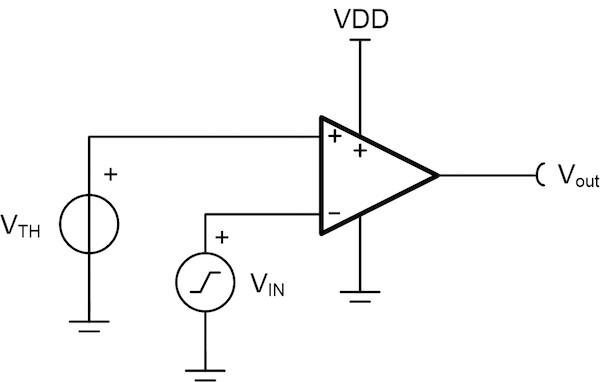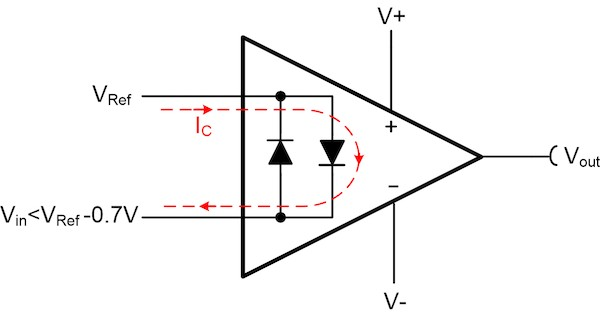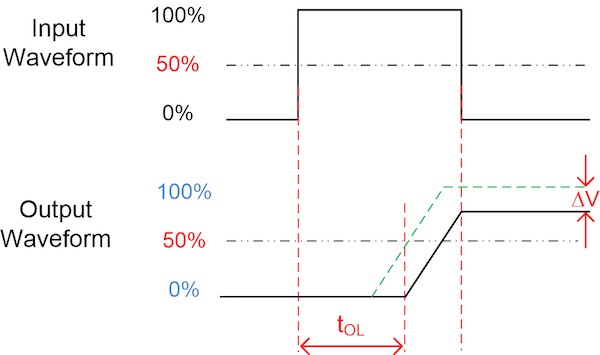### Using Operational Amplifiers as Comparators

• This article discusses the specifications and characteristics to consider when using op-amps as comparators and provides a design procedure.

• Comparators are often used to differentiate between two conditions in a system: For example, outputting a logic high (5V) in an overvoltage situation and a logic low (0V) for normal operation. Along with dedicated comparators, it’s possible to configure operational amplifiers (op-amps) to operate as comparators.

• Op-amps have several benefits over dedicated comparators, costing less and minimizing printed circuit board (PCB) area. However, you must consider multiple op-amp specifications or characteristics before configuring an op-amp as a comparator.

## Design Considerations

• When configuring an op-amp as a comparator, you need to consider the presence of differential input clamping diodes (back-to-back diodes), the input common-mode voltage, the slew rate and the overload recovery time. Figure 1 shows a typical comparator configuration when using an op-amp.

• Figure 1. The typical comparator configuration using an op-amp.## Differential Input Clamping Diodes

• Differential input clamping diodes, also called back-to-back input diodes, protect the input stage transistors from large differential input voltages. Figure 2 shows the internal differential input clamping diodes.

• Figure 2. Input clamping diodes• An op-amp cannot have differential input clamping diodes when used as a comparator. When applying a differential signal larger than a diode drop to an op-amp with differential input clamping diodes, one of the diodes between the noninverting and inverting inputs will conduct, shorting the two inputs together. During this condition, excessive current will flow through the appropriate diode, potentially damaging the device. Figure 3 illustrates this effect when the input diode conducts and current flows from a reference source (VRef) to the input voltage (Vin).

• Figure 3. The clamping diode conducts when the differential input is too large.

​​​​​​​​​​​​​​Input Common-Mode Voltage

• The common-mode voltage range defines the linear operating region of an op-amp’s input stage. The voltage on the op-amp’s inputs should be within this range; otherwise, undesirable results such as phase inversion.

## Propagation Delay

• When configuring an op-amp as a comparator, the propagation delay is the total time for the output voltage to transition from low to high or high to low after the input transitions. The total transition time depends on the op-amp’s overload recovery time and slew rate. Equation 1 calculates the total op-amp output transition time:

• tTOTAL = tOL + tS

• where tOL is the overload recovery time and tS is the time to slew.

• The input voltage should not change until the output settles to its final value. Figure 4 displays a typical output voltage waveform of an op-amp configured as a comparator. Notice that the output voltage completely transitions before the input changes.

• Figure 4. Propagation delay